Скачать презентацию Data Mining Credibility Evaluating What s Been Learned

0e4f6952a7f608a193192521948d5226.ppt

• Количество слайдов: 36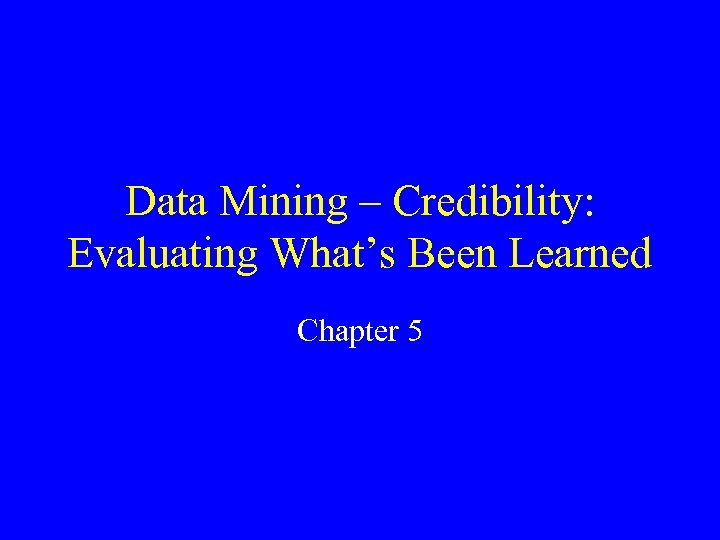Data Mining – Credibility: Evaluating What’s Been Learned Chapter 5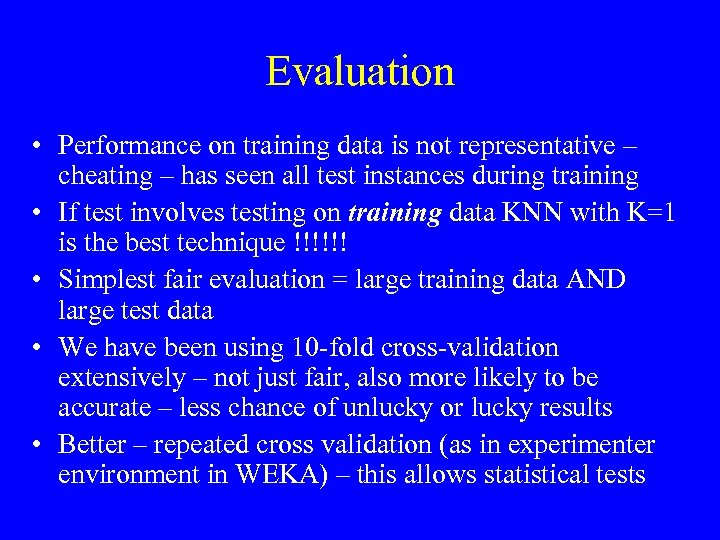Evaluation • Performance on training data is not representative – cheating – has seen all test instances during training • If test involves testing on training data KNN with K=1 is the best technique !!!!!! • Simplest fair evaluation = large training data AND large test data • We have been using 10 -fold cross-validation extensively – not just fair, also more likely to be accurate – less chance of unlucky or lucky results • Better – repeated cross validation (as in experimenter environment in WEKA) – this allows statistical tests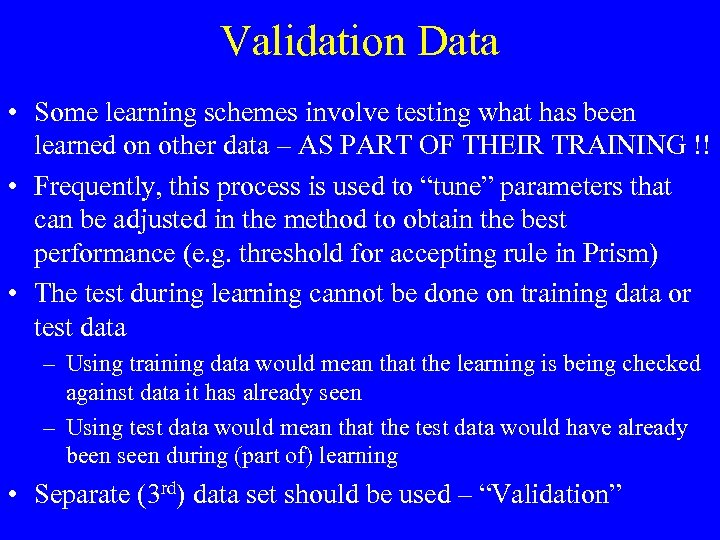Validation Data • Some learning schemes involve testing what has been learned on other data – AS PART OF THEIR TRAINING !! • Frequently, this process is used to “tune” parameters that can be adjusted in the method to obtain the best performance (e. g. threshold for accepting rule in Prism) • The test during learning cannot be done on training data or test data – Using training data would mean that the learning is being checked against data it has already seen – Using test data would mean that the test data would have already been seen during (part of) learning • Separate (3 rd) data set should be used – “Validation”Confidence Intervals • If experiment shows 75% correct, we might be interested in what the correctness rate can actually be expected to be (the experiment is a result of sampling) • We can develop a confidence interval around the result • Skip Math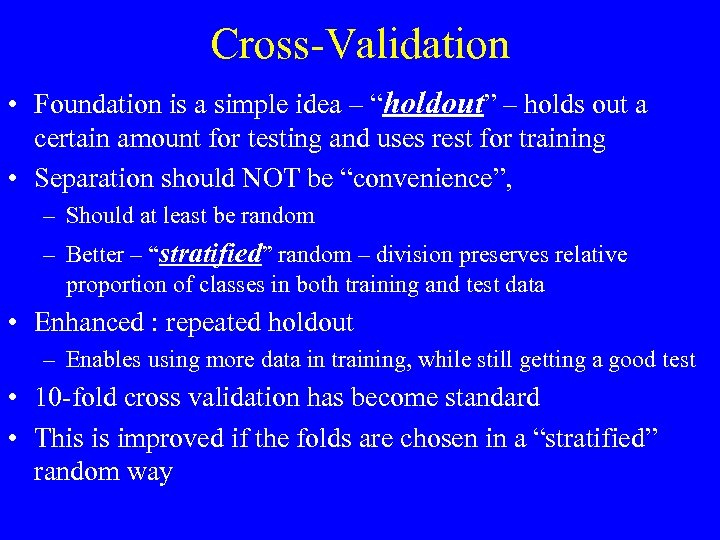Cross-Validation • Foundation is a simple idea – “holdout” – holds out a certain amount for testing and uses rest for training • Separation should NOT be “convenience”, – Should at least be random – Better – “stratified” random – division preserves relative proportion of classes in both training and test data • Enhanced : repeated holdout – Enables using more data in training, while still getting a good test • 10 -fold cross validation has become standard • This is improved if the folds are chosen in a “stratified” random way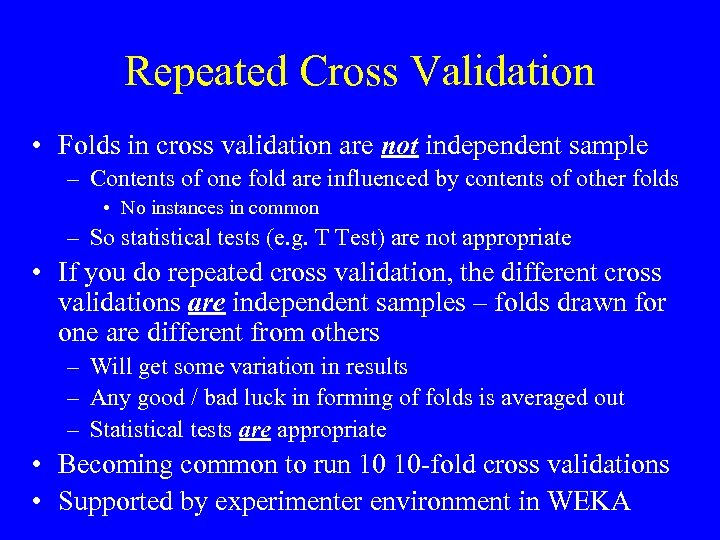Repeated Cross Validation • Folds in cross validation are not independent sample – Contents of one fold are influenced by contents of other folds • No instances in common – So statistical tests (e. g. T Test) are not appropriate • If you do repeated cross validation, the different cross validations are independent samples – folds drawn for one are different from others – Will get some variation in results – Any good / bad luck in forming of folds is averaged out – Statistical tests are appropriate • Becoming common to run 10 10 -fold cross validations • Supported by experimenter environment in WEKA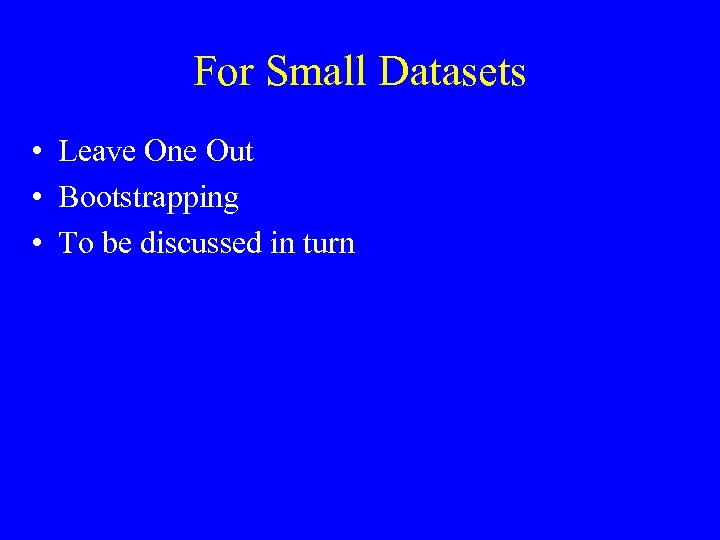For Small Datasets • Leave One Out • Bootstrapping • To be discussed in turn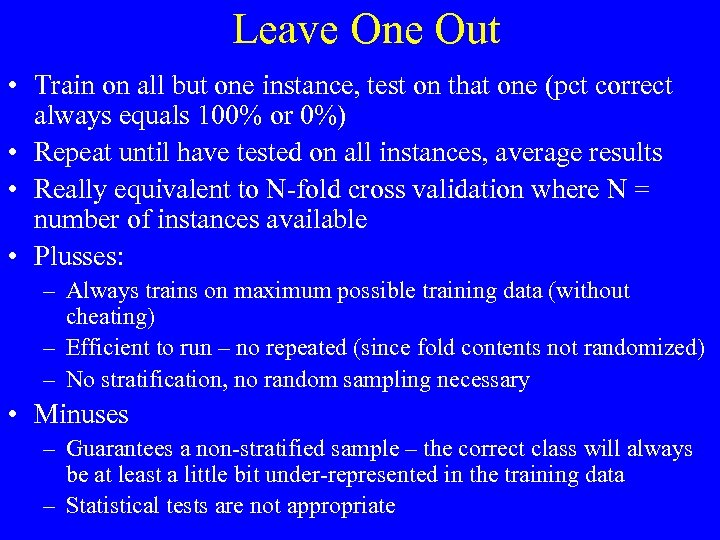Leave One Out • Train on all but one instance, test on that one (pct correct always equals 100% or 0%) • Repeat until have tested on all instances, average results • Really equivalent to N-fold cross validation where N = number of instances available • Plusses: – Always trains on maximum possible training data (without cheating) – Efficient to run – no repeated (since fold contents not randomized) – No stratification, no random sampling necessary • Minuses – Guarantees a non-stratified sample – the correct class will always be at least a little bit under-represented in the training data – Statistical tests are not appropriate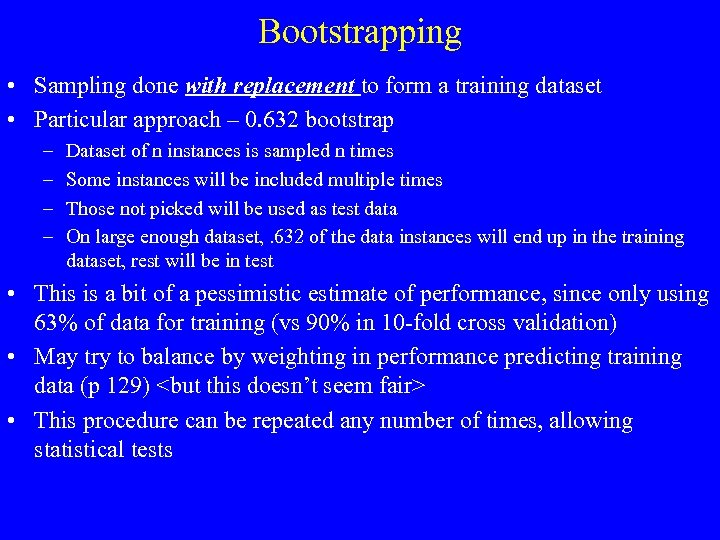Bootstrapping • Sampling done with replacement to form a training dataset • Particular approach – 0. 632 bootstrap – – Dataset of n instances is sampled n times Some instances will be included multiple times Those not picked will be used as test data On large enough dataset, . 632 of the data instances will end up in the training dataset, rest will be in test • This is a bit of a pessimistic estimate of performance, since only using 63% of data for training (vs 90% in 10 -fold cross validation) • May try to balance by weighting in performance predicting training data (p 129) • This procedure can be repeated any number of times, allowing statistical tests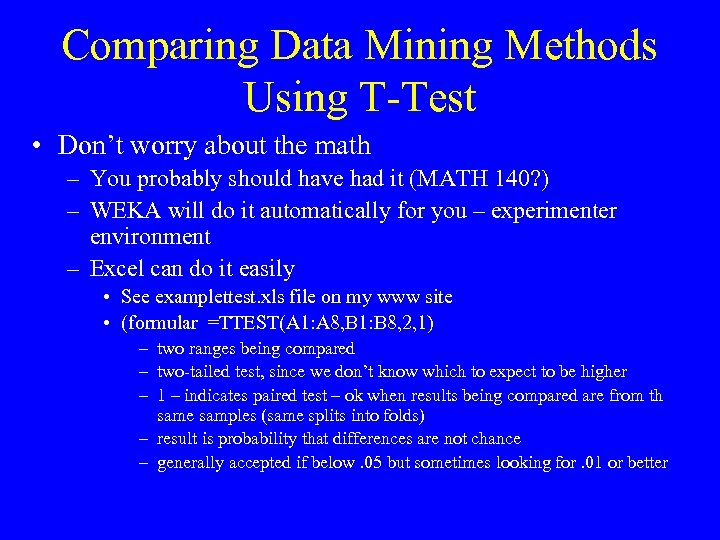Comparing Data Mining Methods Using T-Test • Don’t worry about the math – You probably should have had it (MATH 140? ) – WEKA will do it automatically for you – experimenter environment – Excel can do it easily • See examplettest. xls file on my www site • (formular =TTEST(A 1: A 8, B 1: B 8, 2, 1) – two ranges being compared – two-tailed test, since we don’t know which to expect to be higher – 1 – indicates paired test – ok when results being compared are from th same samples (same splits into folds) – result is probability that differences are not chance – generally accepted if below. 05 but sometimes looking for. 01 or better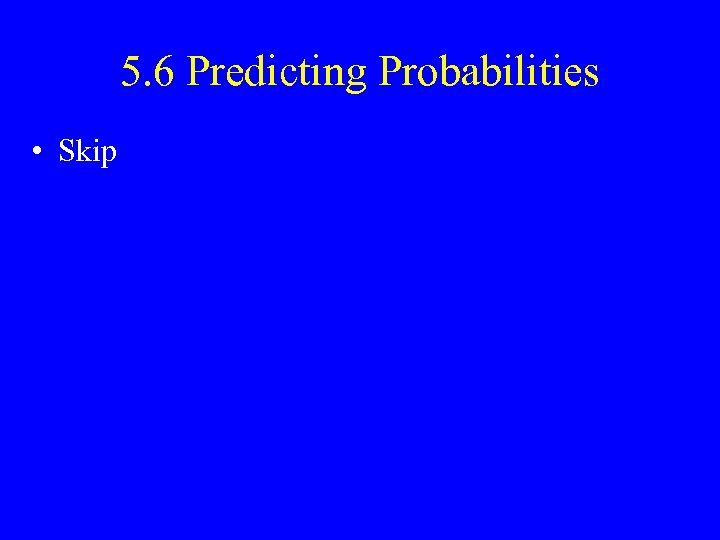5. 6 Predicting Probabilities • Skip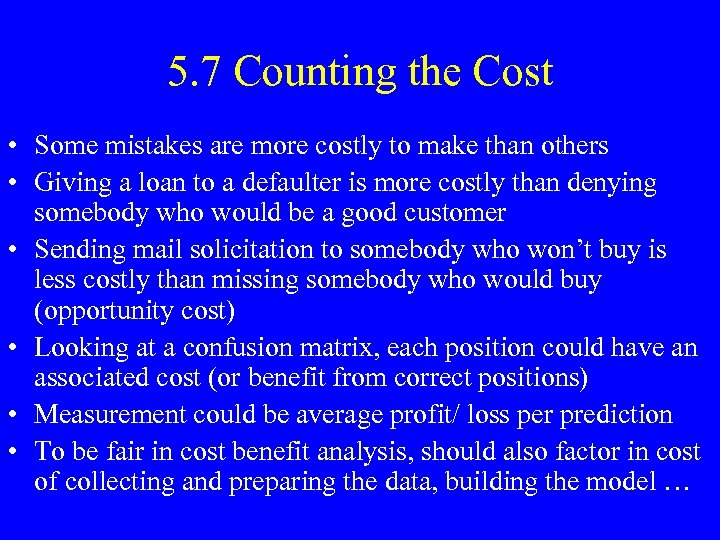5. 7 Counting the Cost • Some mistakes are more costly to make than others • Giving a loan to a defaulter is more costly than denying somebody who would be a good customer • Sending mail solicitation to somebody who won’t buy is less costly than missing somebody who would buy (opportunity cost) • Looking at a confusion matrix, each position could have an associated cost (or benefit from correct positions) • Measurement could be average profit/ loss per prediction • To be fair in cost benefit analysis, should also factor in cost of collecting and preparing the data, building the model …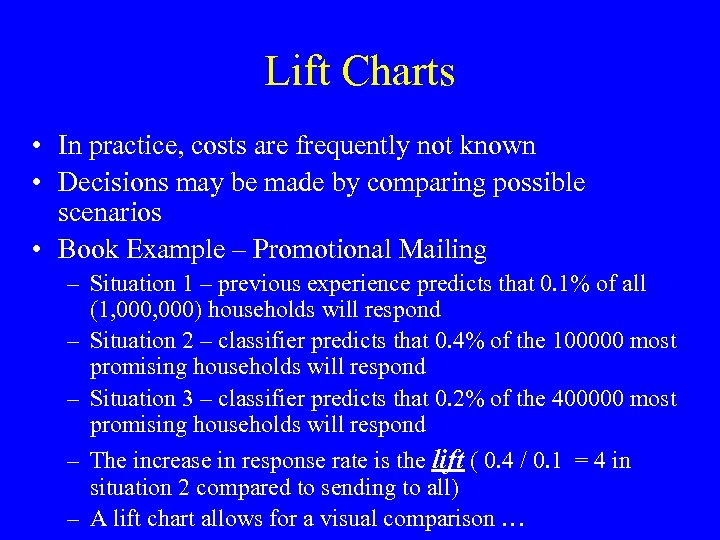Lift Charts • In practice, costs are frequently not known • Decisions may be made by comparing possible scenarios • Book Example – Promotional Mailing – Situation 1 – previous experience predicts that 0. 1% of all (1, 000) households will respond – Situation 2 – classifier predicts that 0. 4% of the 100000 most promising households will respond – Situation 3 – classifier predicts that 0. 2% of the 400000 most promising households will respond – The increase in response rate is the lift ( 0. 4 / 0. 1 = 4 in situation 2 compared to sending to all) – A lift chart allows for a visual comparison …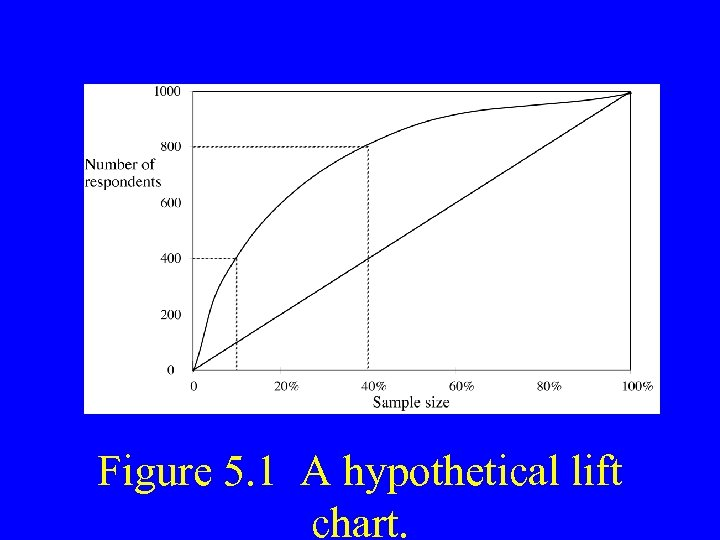Figure 5. 1 A hypothetical lift chart.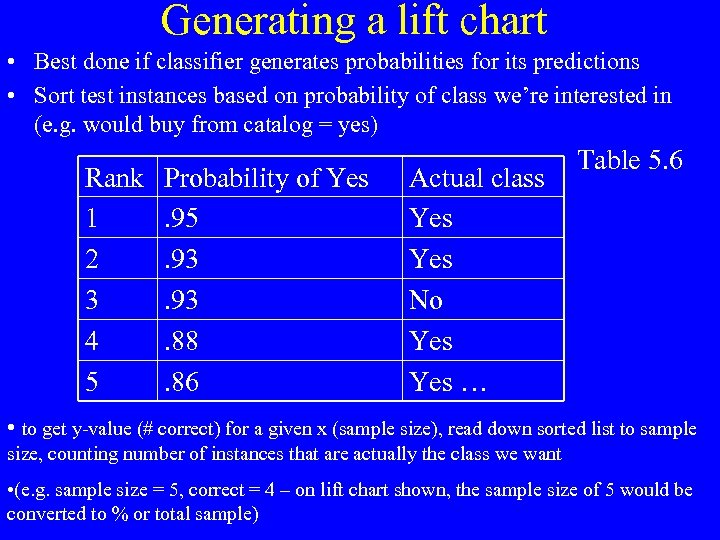Generating a lift chart • Best done if classifier generates probabilities for its predictions • Sort test instances based on probability of class we’re interested in (e. g. would buy from catalog = yes) Rank 1 2 3 4 5 Probability of Yes. 95. 93. 88. 86 Actual class Yes No Yes … Table 5. 6 • to get y-value (# correct) for a given x (sample size), read down sorted list to sample size, counting number of instances that are actually the class we want • (e. g. sample size = 5, correct = 4 – on lift chart shown, the sample size of 5 would be converted to % or total sample)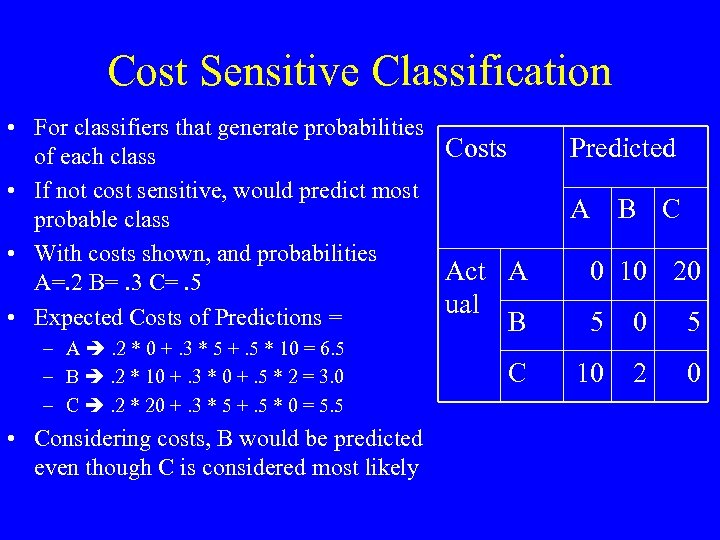Cost Sensitive Classification • For classifiers that generate probabilities Costs of each class • If not cost sensitive, would predict most probable class • With costs shown, and probabilities Act A A=. 2 B=. 3 C=. 5 ual • Expected Costs of Predictions = B – A . 2 * 0 +. 3 * 5 +. 5 * 10 = 6. 5 – B . 2 * 10 +. 3 * 0 +. 5 * 2 = 3. 0 – C . 2 * 20 +. 3 * 5 +. 5 * 0 = 5. 5 • Considering costs, B would be predicted even though C is considered most likely C Predicted A B C 0 10 20 5 10 2 0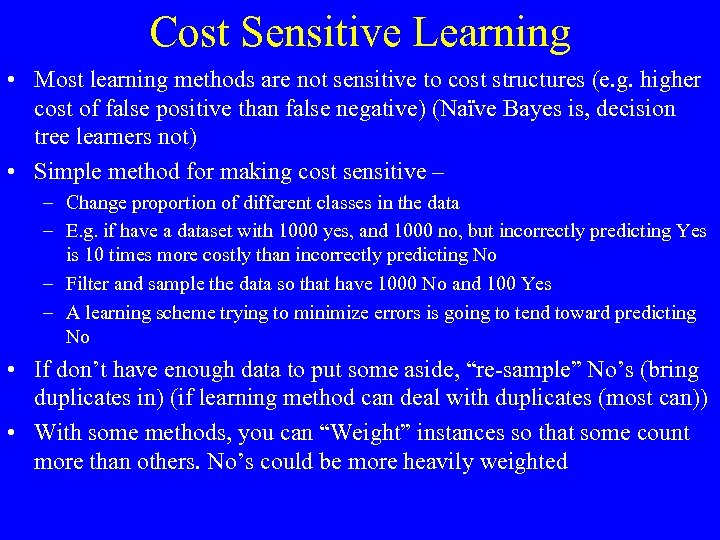Cost Sensitive Learning • Most learning methods are not sensitive to cost structures (e. g. higher cost of false positive than false negative) (Naïve Bayes is, decision tree learners not) • Simple method for making cost sensitive – – Change proportion of different classes in the data – E. g. if have a dataset with 1000 yes, and 1000 no, but incorrectly predicting Yes is 10 times more costly than incorrectly predicting No – Filter and sample the data so that have 1000 No and 100 Yes – A learning scheme trying to minimize errors is going to tend toward predicting No • If don’t have enough data to put some aside, “re-sample” No’s (bring duplicates in) (if learning method can deal with duplicates (most can)) • With some methods, you can “Weight” instances so that some count more than others. No’s could be more heavily weighted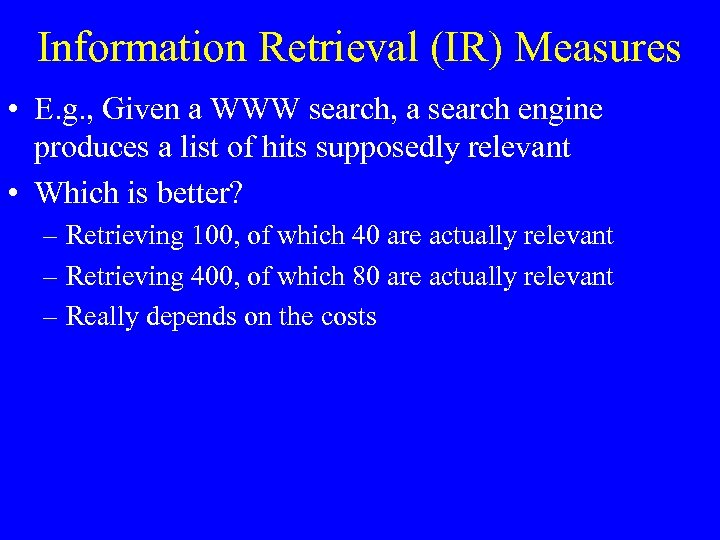Information Retrieval (IR) Measures • E. g. , Given a WWW search, a search engine produces a list of hits supposedly relevant • Which is better? – Retrieving 100, of which 40 are actually relevant – Retrieving 400, of which 80 are actually relevant – Really depends on the costs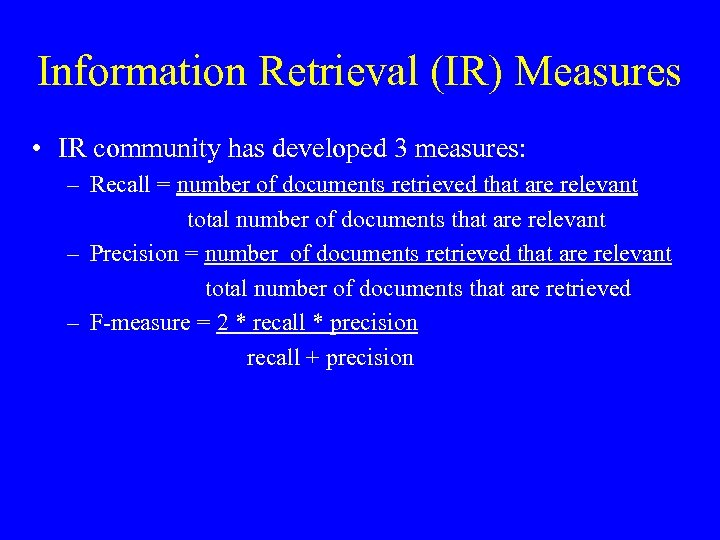Information Retrieval (IR) Measures • IR community has developed 3 measures: – Recall = number of documents retrieved that are relevant total number of documents that are relevant – Precision = number of documents retrieved that are relevant total number of documents that are retrieved – F-measure = 2 * recall * precision recall + precision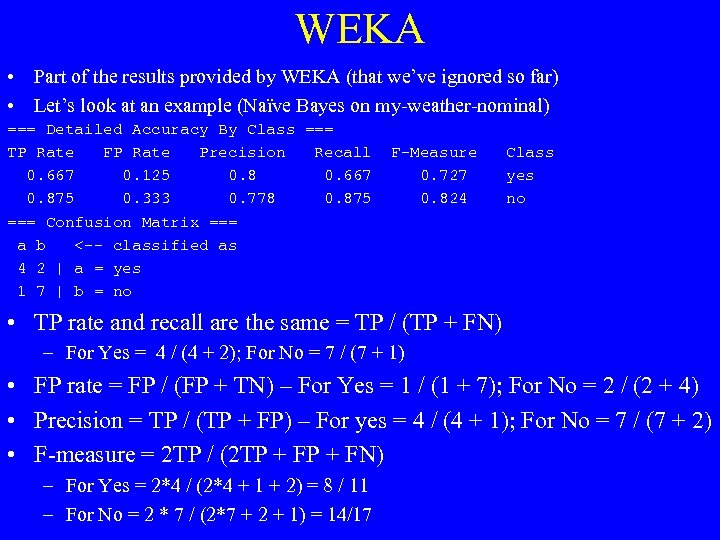WEKA • Part of the results provided by WEKA (that we’ve ignored so far) • Let’s look at an example (Naïve Bayes on my-weather-nominal) === Detailed Accuracy By Class === TP Rate FP Rate Precision Recall 0. 667 0. 125 0. 8 0. 667 0. 875 0. 333 0. 778 0. 875 === Confusion Matrix === a b <-- classified as 4 2 | a = yes 1 7 | b = no F-Measure 0. 727 0. 824 Class yes no • TP rate and recall are the same = TP / (TP + FN) – For Yes = 4 / (4 + 2); For No = 7 / (7 + 1) • FP rate = FP / (FP + TN) – For Yes = 1 / (1 + 7); For No = 2 / (2 + 4) • Precision = TP / (TP + FP) – For yes = 4 / (4 + 1); For No = 7 / (7 + 2) • F-measure = 2 TP / (2 TP + FN) – For Yes = 2*4 / (2*4 + 1 + 2) = 8 / 11 – For No = 2 * 7 / (2*7 + 2 + 1) = 14/17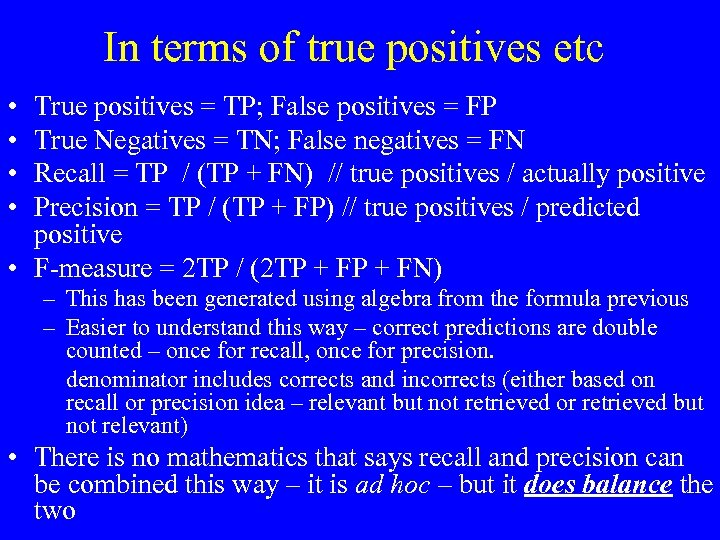In terms of true positives etc • • True positives = TP; False positives = FP True Negatives = TN; False negatives = FN Recall = TP / (TP + FN) // true positives / actually positive Precision = TP / (TP + FP) // true positives / predicted positive • F-measure = 2 TP / (2 TP + FN) – This has been generated using algebra from the formula previous – Easier to understand this way – correct predictions are double counted – once for recall, once for precision. denominator includes corrects and incorrects (either based on recall or precision idea – relevant but not retrieved or retrieved but not relevant) • There is no mathematics that says recall and precision can be combined this way – it is ad hoc – but it does balance the two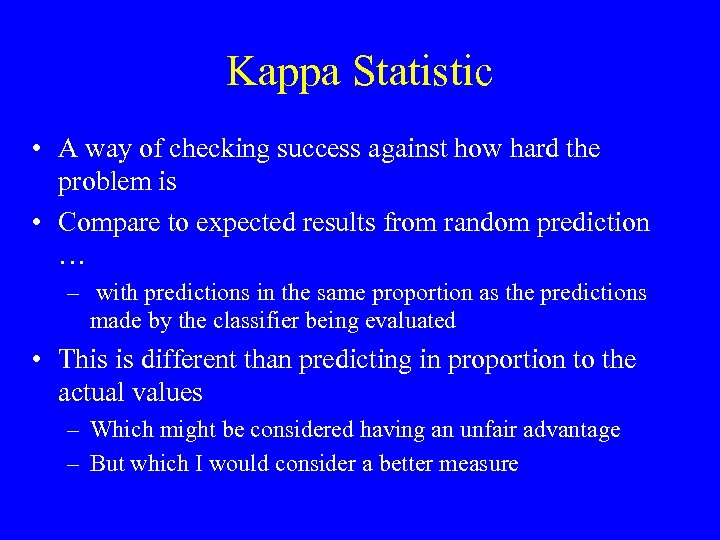Kappa Statistic • A way of checking success against how hard the problem is • Compare to expected results from random prediction … – with predictions in the same proportion as the predictions made by the classifier being evaluated • This is different than predicting in proportion to the actual values – Which might be considered having an unfair advantage – But which I would consider a better measure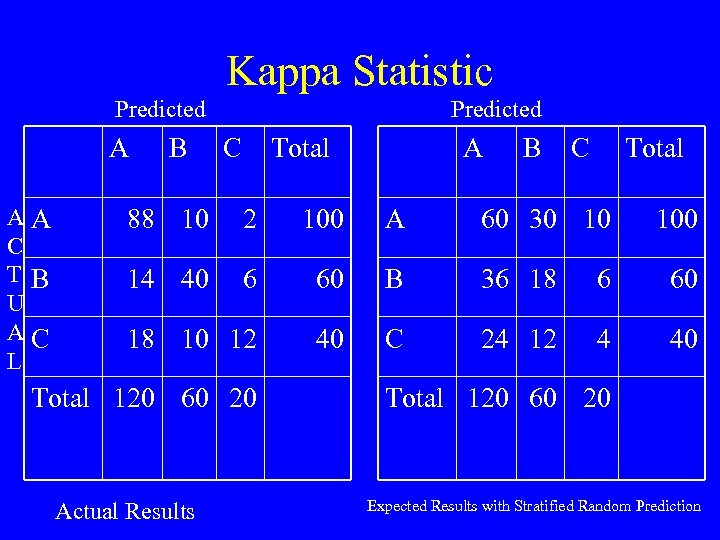Kappa Statistic Predicted A AA C TB U AC L B Predicted C Total A B C Total 88 10 2 100 A 60 30 10 14 40 6 60 B 36 18 6 60 18 10 12 40 C 24 12 4 40 Total 120 60 20 Actual Results 100 Total 120 60 20 Expected Results with Stratified Random Prediction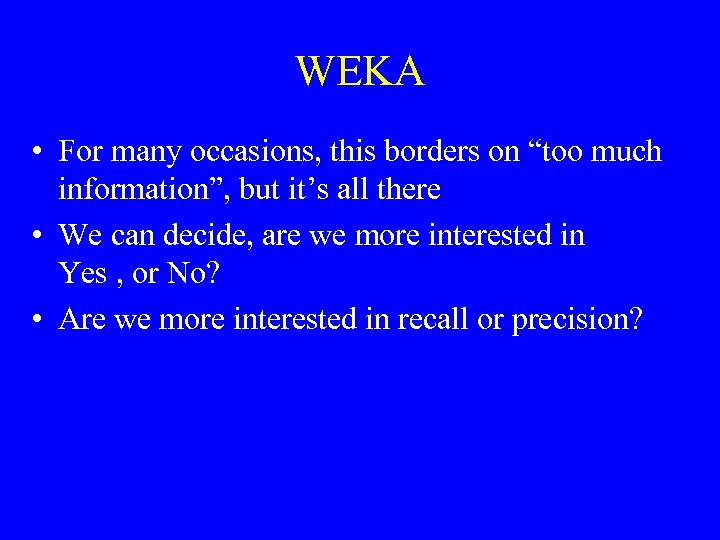WEKA • For many occasions, this borders on “too much information”, but it’s all there • We can decide, are we more interested in Yes , or No? • Are we more interested in recall or precision?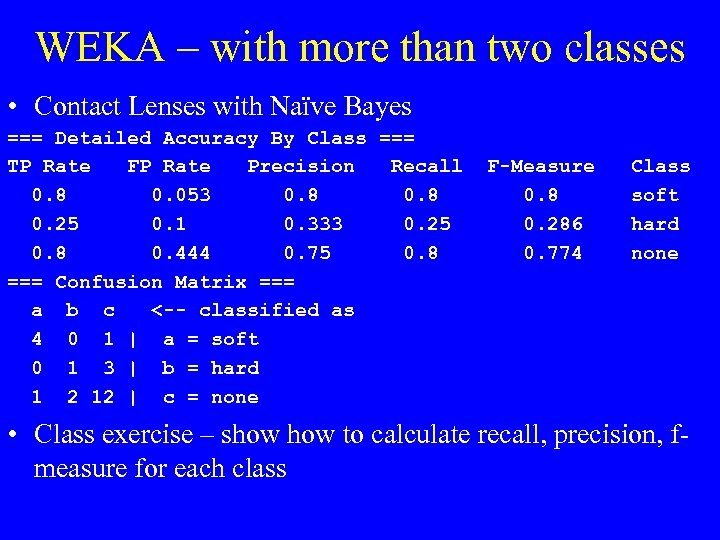WEKA – with more than two classes • Contact Lenses with Naïve Bayes === Detailed Accuracy By Class === TP Rate FP Rate Precision Recall 0. 8 0. 053 0. 8 0. 25 0. 1 0. 333 0. 25 0. 8 0. 444 0. 75 0. 8 === Confusion Matrix === a b c <-- classified as 4 0 1 | a = soft 0 1 3 | b = hard 1 2 12 | c = none F-Measure 0. 8 0. 286 0. 774 Class soft hard none • Class exercise – show to calculate recall, precision, fmeasure for each class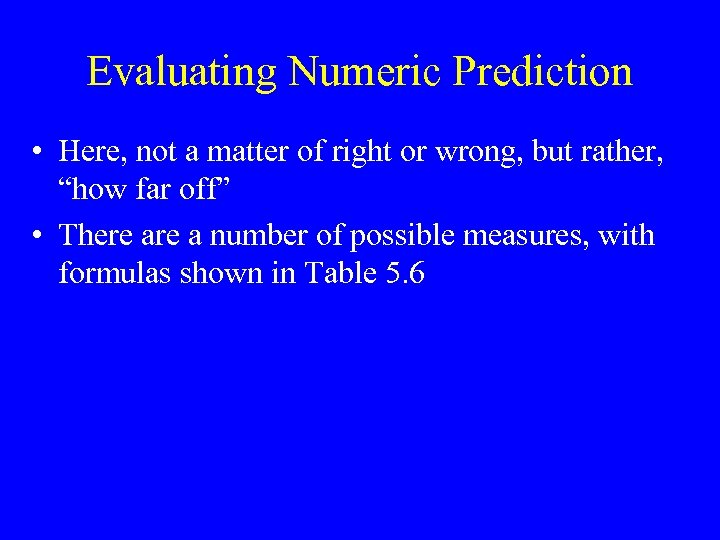Evaluating Numeric Prediction • Here, not a matter of right or wrong, but rather, “how far off” • There a number of possible measures, with formulas shown in Table 5. 6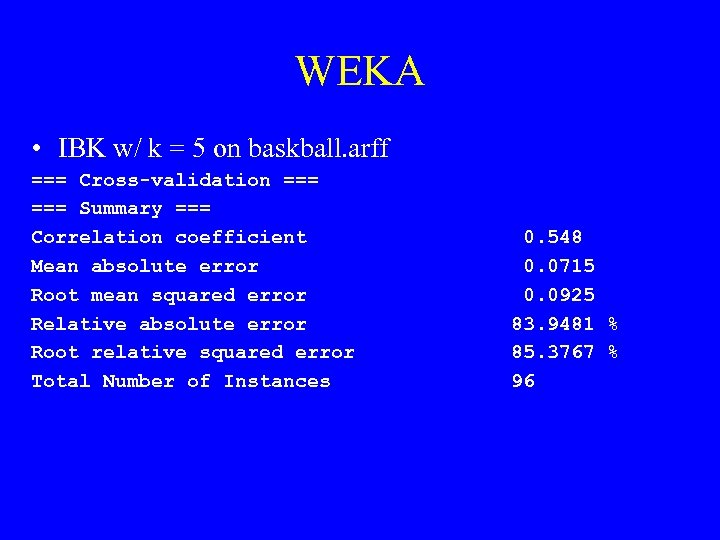WEKA • IBK w/ k = 5 on baskball. arff === Cross-validation === Summary === Correlation coefficient Mean absolute error Root mean squared error Relative absolute error Root relative squared error Total Number of Instances 0. 548 0. 0715 0. 0925 83. 9481 % 85. 3767 % 96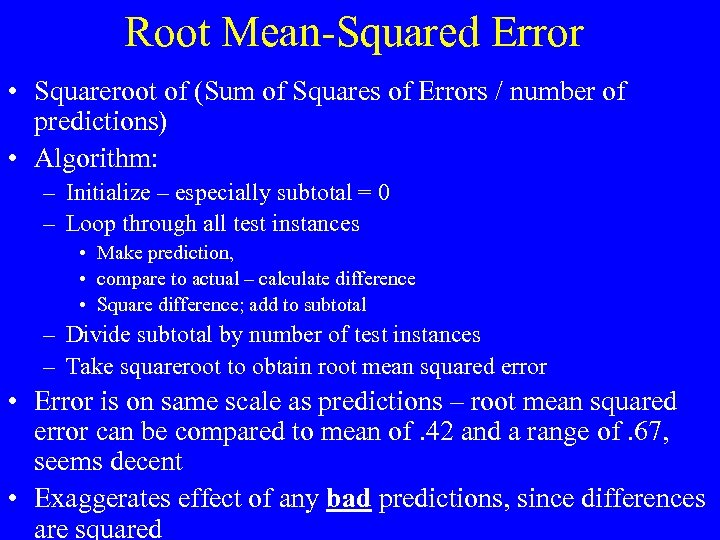Root Mean-Squared Error • Squareroot of (Sum of Squares of Errors / number of predictions) • Algorithm: – Initialize – especially subtotal = 0 – Loop through all test instances • Make prediction, • compare to actual – calculate difference • Square difference; add to subtotal – Divide subtotal by number of test instances – Take squareroot to obtain root mean squared error • Error is on same scale as predictions – root mean squared error can be compared to mean of. 42 and a range of. 67, seems decent • Exaggerates effect of any bad predictions, since differences are squared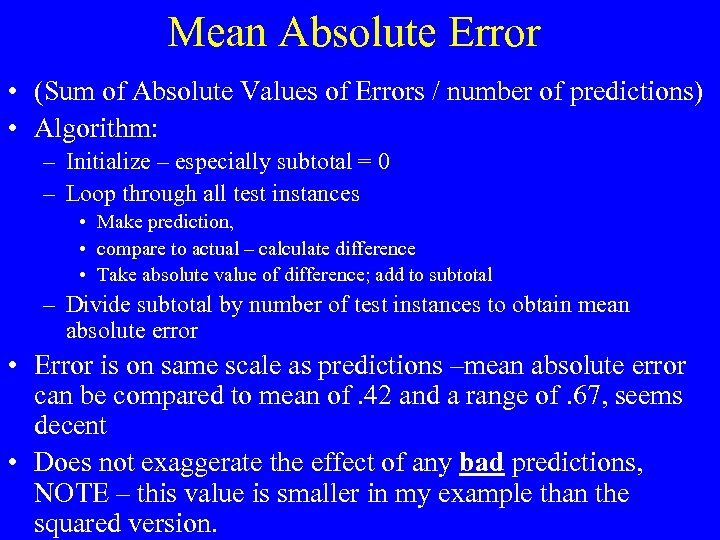Mean Absolute Error • (Sum of Absolute Values of Errors / number of predictions) • Algorithm: – Initialize – especially subtotal = 0 – Loop through all test instances • Make prediction, • compare to actual – calculate difference • Take absolute value of difference; add to subtotal – Divide subtotal by number of test instances to obtain mean absolute error • Error is on same scale as predictions –mean absolute error can be compared to mean of. 42 and a range of. 67, seems decent • Does not exaggerate the effect of any bad predictions, NOTE – this value is smaller in my example than the squared version.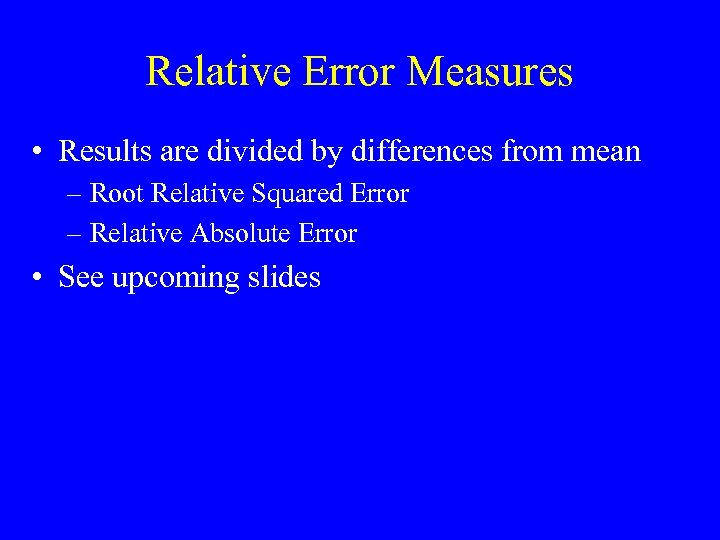Relative Error Measures • Results are divided by differences from mean – Root Relative Squared Error – Relative Absolute Error • See upcoming slides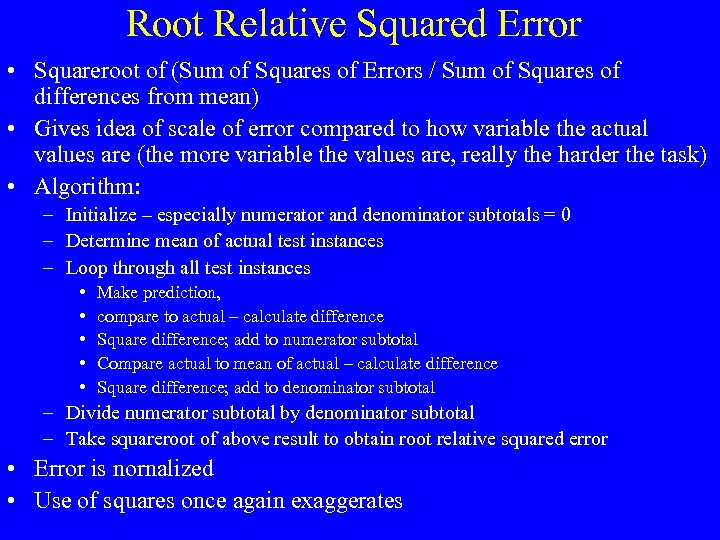Root Relative Squared Error • Squareroot of (Sum of Squares of Errors / Sum of Squares of differences from mean) • Gives idea of scale of error compared to how variable the actual values are (the more variable the values are, really the harder the task) • Algorithm: – Initialize – especially numerator and denominator subtotals = 0 – Determine mean of actual test instances – Loop through all test instances • • • Make prediction, compare to actual – calculate difference Square difference; add to numerator subtotal Compare actual to mean of actual – calculate difference Square difference; add to denominator subtotal – Divide numerator subtotal by denominator subtotal – Take squareroot of above result to obtain root relative squared error • Error is nornalized • Use of squares once again exaggerates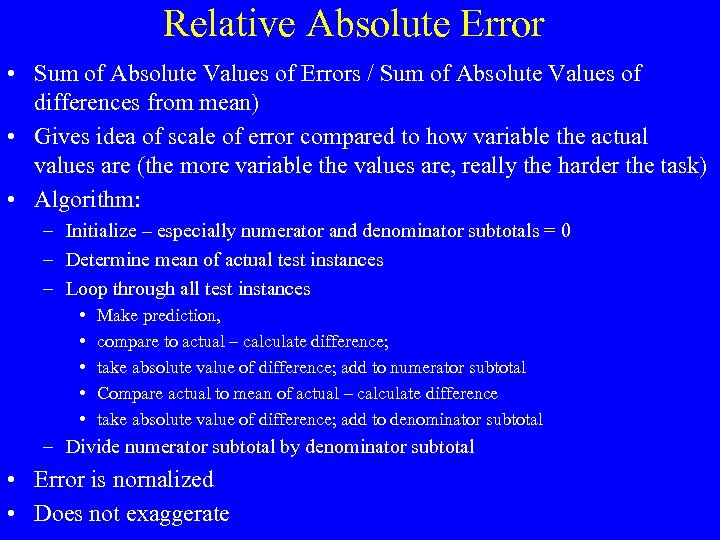Relative Absolute Error • Sum of Absolute Values of Errors / Sum of Absolute Values of differences from mean) • Gives idea of scale of error compared to how variable the actual values are (the more variable the values are, really the harder the task) • Algorithm: – Initialize – especially numerator and denominator subtotals = 0 – Determine mean of actual test instances – Loop through all test instances • • • Make prediction, compare to actual – calculate difference; take absolute value of difference; add to numerator subtotal Compare actual to mean of actual – calculate difference take absolute value of difference; add to denominator subtotal – Divide numerator subtotal by denominator subtotal • Error is nornalized • Does not exaggerate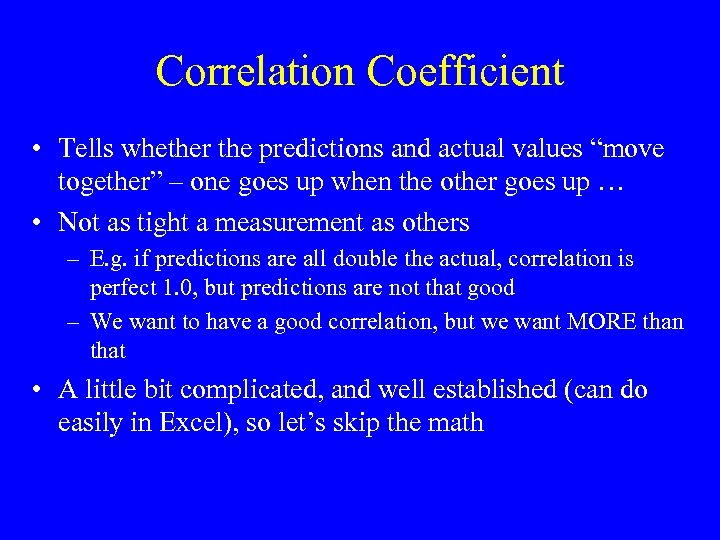Correlation Coefficient • Tells whether the predictions and actual values “move together” – one goes up when the other goes up … • Not as tight a measurement as others – E. g. if predictions are all double the actual, correlation is perfect 1. 0, but predictions are not that good – We want to have a good correlation, but we want MORE than that • A little bit complicated, and well established (can do easily in Excel), so let’s skip the math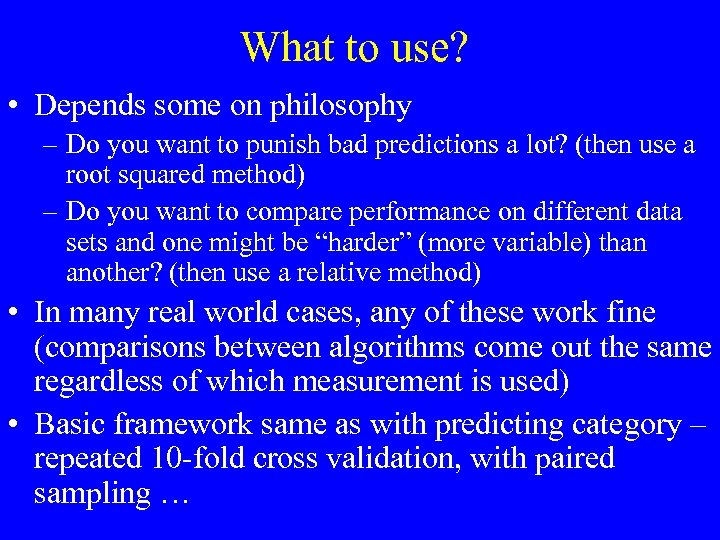What to use? • Depends some on philosophy – Do you want to punish bad predictions a lot? (then use a root squared method) – Do you want to compare performance on different data sets and one might be “harder” (more variable) than another? (then use a relative method) • In many real world cases, any of these work fine (comparisons between algorithms come out the same regardless of which measurement is used) • Basic framework same as with predicting category – repeated 10 -fold cross validation, with paired sampling …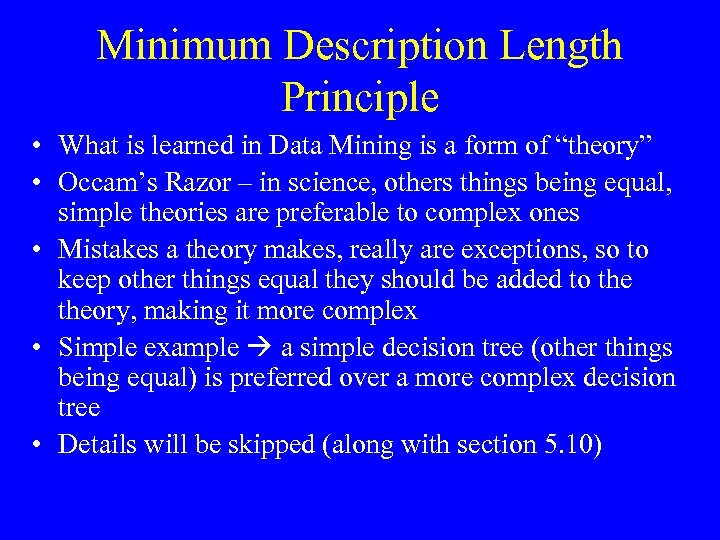Minimum Description Length Principle • What is learned in Data Mining is a form of “theory” • Occam’s Razor – in science, others things being equal, simple theories are preferable to complex ones • Mistakes a theory makes, really are exceptions, so to keep other things equal they should be added to theory, making it more complex • Simple example a simple decision tree (other things being equal) is preferred over a more complex decision tree • Details will be skipped (along with section 5. 10)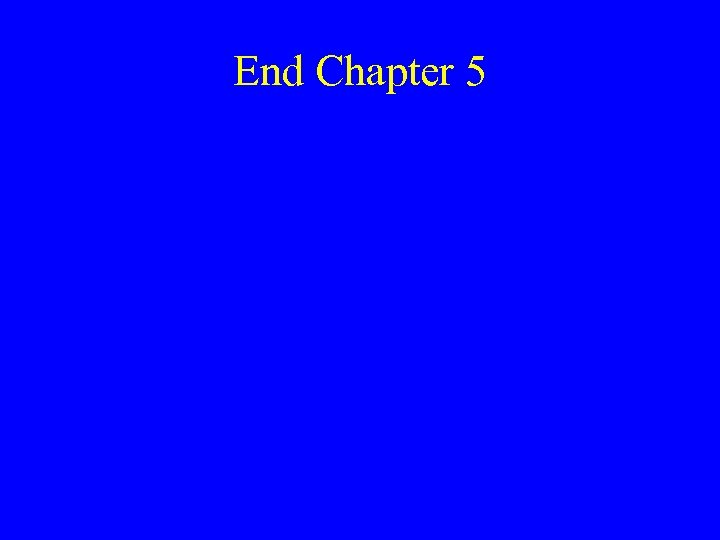End Chapter 5